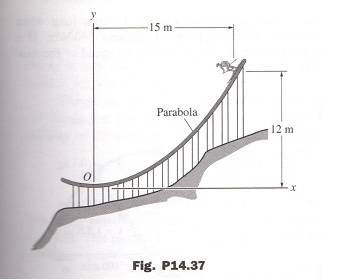# Calculate the contact force between the skis and the track

## Homework Statement

14.37 A 75-kg ski jumper starts his run in the position shown on the parabolic
track. The equation of the parabola is ##y = (12/225)x^2##, where x and y are in meters.
Neglecting friction, calculate the contact force between the skis and the track at O.## The Attempt at a Solution

[/B]
At pont A (start of run):

##Vg_A=mg(12)##
##T_A=0##

At pont O:

##Vg_O = 0##
##T_O=1/2mv_O^2##

Something is missing?
Any hints how to continue?

## Answers and Replies

BvU
Science Advisor
Homework Helper
Something is missing?
Indeed ! Some relevant equations featuring the variables and given information !

haruspex
Science Advisor
Homework Helper
Gold Member
2020 Award
how to continue?
So can you find the velocity at O?
What else do you need to know to figure out the force there?